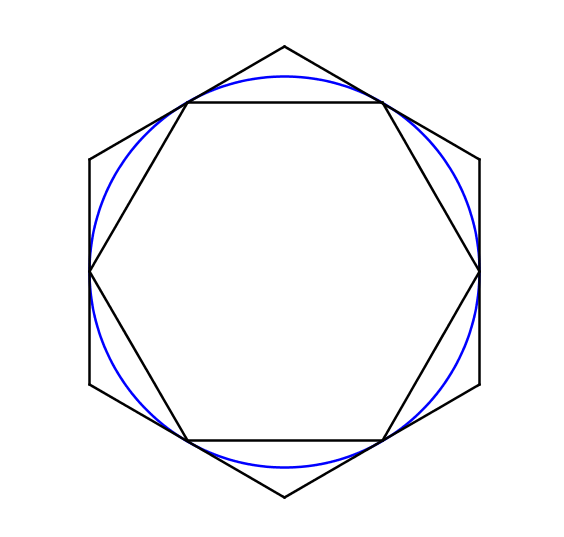# Approximating $\pi$ with hexagonsIn the figure to the right, a circle is circumscribed around a regular hexagon, and the same circle is inscribed within another regular hexagon.

Let $P_1$ be the perimeter of the larger hexagon, let $P_2$ be the perimeter of the smaller hexagon, and let $C$ be the circumference of the circle.

The circumference of the circle can be approximated by finding the mean of the two perimeters:

$C\approx \frac{P_1+P_2}{2}.$

If $\pi$ is approximated using the approximation for circumference above, then $\pi\approx\frac{a}{b}+\sqrt{c}$, where $a, b, c$ are positive integers, $a$ and $b$ are coprime, and $c$ is square-free.

Find $a+b+c$.

×